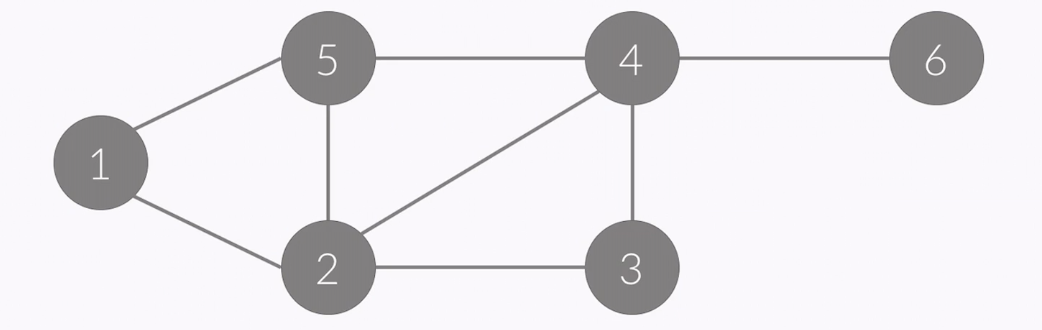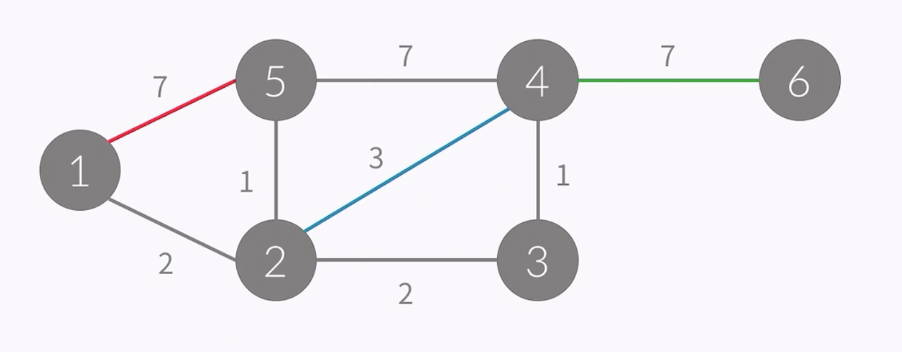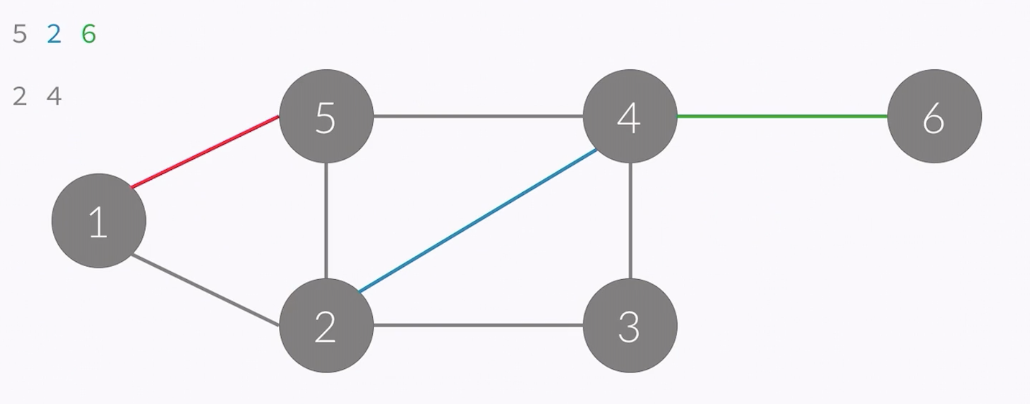# Algorithm Study #6 (Graph_Expression_How_To_Save_Graph)

jakeseo_me·2019년 3월 12일
0

목록 보기
16/18

## Representation of Graph• in case of this graph, it has 6 vertices and 8 edges
• it doesn't have direction so it is undirected graph
• vertices : {1, 2, 3, 4, 5, 6}
• edges : {(1, 2), (1, 5), (2, 5), (2, 3), (3, 4), (2, 4), (4, 5), (4, 6)}
• vertices are usually named just 1 to the amount of vertices
• so edges are important
• we only save the amount of vertices by using variable.
• if we just want to express graph, it is the same to just save the all of edges.
• edge can save effectively
- we can figure out the graph's representation

• When you say the number of vertices is V
• We use 2 dimension array which has size of V * V
• A[i][j] = 1 (when there is edge connecting i to j), 0 (no edge)
• in case of graph at the top, it can be expressed like this
/ 1 2 3 4 5 6
1 0 0 0 0 1 0
2 1 0 1 1 1 0
3 0 1 0 1 0 0
4 0 1 1 0 1 1
5 1 1 0 1 0 0
6 0 0 0 1 0 0
• These numbers are symmetrical in relation of the diagonal
• it saves useless edges
- even if it doesn't have any edge, it saves 0 in that space
• usually, V^2 >= E
• to solve easy problems, it is good way to express graph data structure.
#include <cstdio>
#include <vector>
int a;
int main() {
int n, m;
scanf("%d %d", &n, &m);
for (int i=0; i<m; i++){
int u, v;
scanf("%d %d", &u, &v);
a[u][v] = a[v][u] = 1; // it means undirected graph
}
}• it is similar to that of undirected grpah
• but when it is saved it doesn't just save 1 but its weight
• A[i][j] = w (there is edge connecting from i to j), 0 (no edge)
• if range of w is -9999 <= w <= 9999, we can make 2 arrays.
• one expresses edges connecting vertices
• another expresses weight of edges
• graph above can be expressed like this.
/ 1 2 3 4 5 6
1 0 2 0 0 7 0
2 2 0 2 3 1 0
3 0 2 0 1 0 0
4 0 3 1 0 7 7
5 7 1 0 7 0 0
6 0 0 0 7 0 0
• code
#include <cstdio>
#include <vector>
int a;
int main() {
int n, m;
scanf("%d %d", &n, &m);
for (int i=0; i<m; i++){
int u, v;
scanf("%d %d", &u, &v);
a[u][v] = a[v][u] = 1; // it means undirected graph
}
}

• in A[i], there are linked lists which is connected with 'i'• in this case,
• A: 2 5
• A: 1 3 4 5
• A: 2 4
• A: 3 5 2 6
• A: 1 2 4
• A: 4
• the numbers in array actually doesn't mean vertex but edges
• the amount of its numbers means 'degree'
• it needs space of O(E)
• since LinkedList takes too much time to implement, it is usually implemented with vector in which length can be changed
• it is used to use space only needed
#include <cstdio>
#include <vector>
using namespace std;
vector<int> a; // it is different from expression like a(10)
// a means 10 of 2 dimension array which has changable size
int main() {
int n, m;
scanf("%d %d", &n, &m);
for (int i=0; i<m; i++) {
int u, v;
scanf("%d %d", &u, &v);
a[u].push_back(v); // it means undirected graph
a[v].push_back(u);
}
}

• it saves edges and weight like below
• A: (2, 2) (5, 7)
• A: (1, 2) (3, 2) (4, 3) (5, 1)
• A: (2, 2) (4, 1)
• A: (3, 1) (5, 7) (2, 3) (6, 7)
• A: (1, 7) (2, 1) (4, 7)
• A: (4, 7)
• implementation
#include <cstdio>
#include <vector>
using namespace std;
vector<pair<int, int>> a;
int main() {
int n, m;
scanf("%d %d", &n, &m);
for (int i=0; i<m; i++) {
int u, v, w;
scanf("%d %d %d", &u, &v, &w);
a[u].push_back(make_pair(v, w));
a[v].push_back(make_pair(u, w));
}
}

## Space Complexity of Adjacency Matrix and List

• in most cases, we don't need much space for edges
• so adjacency list is usually right choice to use

## Edge-list

• it is implemented by using array
• it saves all of edges
• for example)
• E = 1 2
• E = 1 5
• E = 2 3
• ....
• each means start point of edge and end point of edge
• if there are 8 edges and the graph is undirected, to implement this, we need 16 spaces
• it should be sorted start point of edge first
• after sorting there should be array like this.
• i .... 0 1 2 3 4 5 6
• cnt[i] 0 2 4 2 4 3 1
• it means the number of edges in the graph
• implementation
for (int i=0; i<m; i++) {
cnt[e[i]] += 1;
}
• after getting the number of all edges to N
• accumulate like this again
• i .... 0 1 2 3 4 5 6
• cnt[i] 0 2 6 8 12 15 16
• implementation
for (int i=1; i<=n; i++) {
cnt[i] = cnt[i-1] + cnt[i];
}
• after this, amazing thing happens
• the range from cnt[i-1] to cnt[i]-1 means that E[cnt[i-1] to E[cnt[i]-1] is the range of the edge number i
• for example)
- edges of vertex 1 exists from E to E
- 0 = cnt[i-1], 1 = cnt[i]-1

## meaning of Saving Graph

• it means we want to save edges
• there are three ways
- ineffective